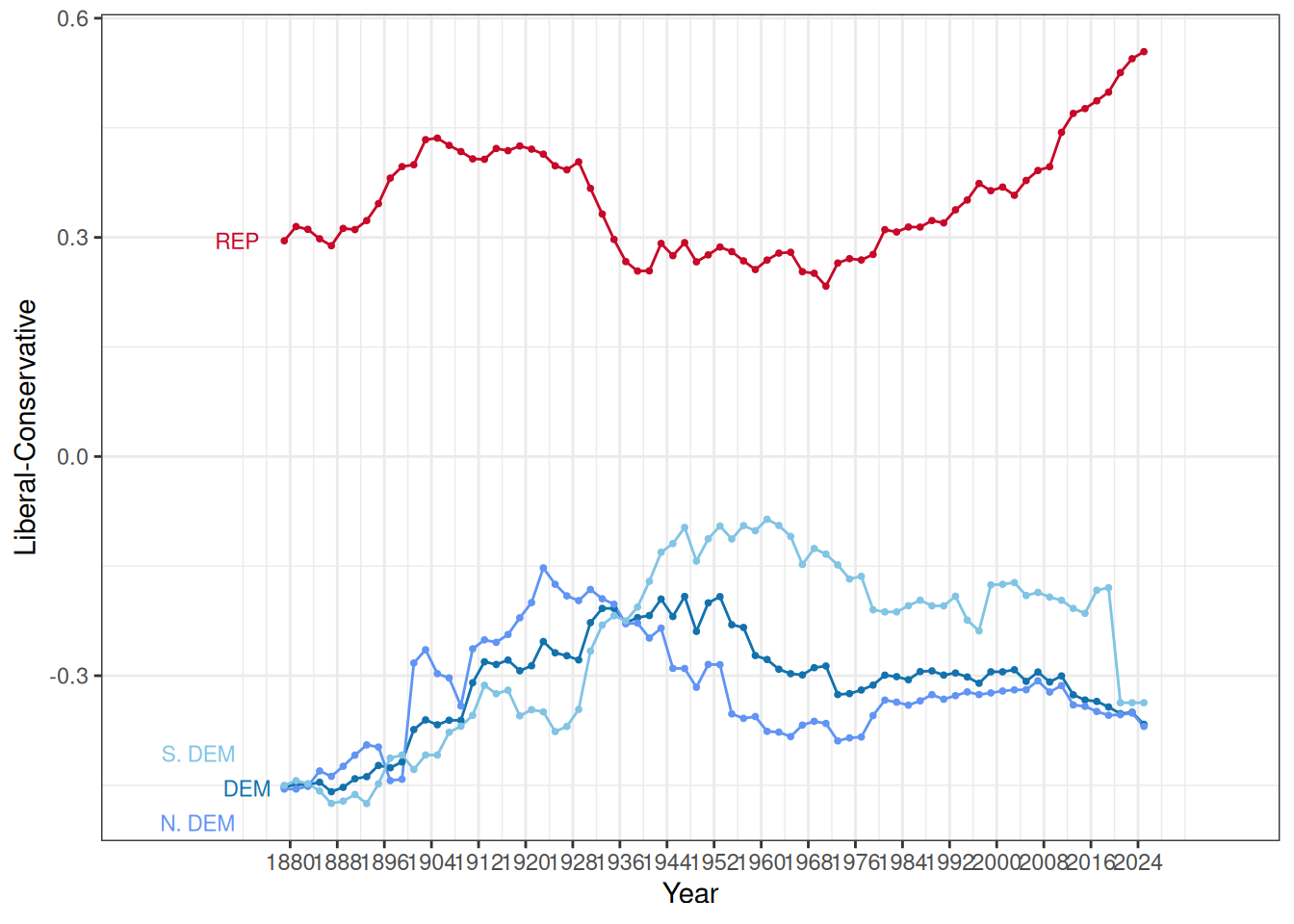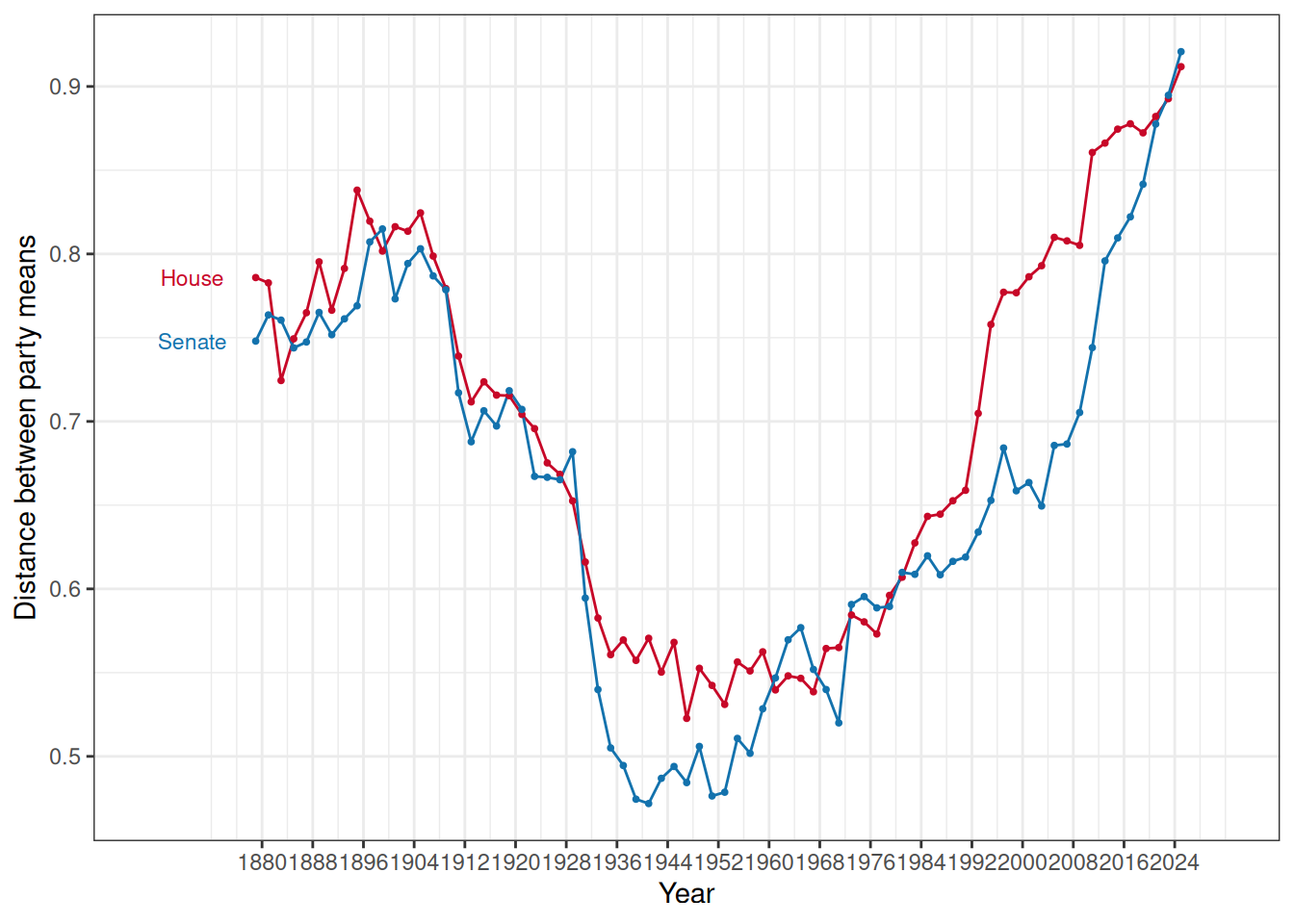# UCLA Presents voteview.combeta

### Polarization in Congress

#### October 23, 2023

Here we create the polarization data and key graphics the Keith shows and describes in greater detail here. You can download the Rmarkdown code that produces this file and all of the data sets and graphics presented here.

This polarization data set includes the following fields (again following Keith):

 0.  Chamber
1.  Congress Number
2.  First Year of the Congress
3.  Difference in Party Means - first dimension
4.  Proportion Moderates
5.  Proportion of moderate Democrats (-0.25 to +0.25)
6.  Proportion of moderate Republicans (-0.25 to +0.25)
7.  Overlap
8.  Chamber Mean - first dimension
9.  Chamber Mean - second dimension
10.  Democratic Party Mean - first dimension
11.  Democratic Party Mean - second dimension
12.  Republican Party Mean - first dimension
13.  Republican Party Mean - second dimension
14.  Northern Republican Mean - first dimension
15.  Northern Republican Mean - second dimension
16.  Southern Republican Mean - first dimension
17.  Southern Republican Mean - second dimension
18.  Northern Democrat Mean - first dimension
19.  Northern Democrat Mean - second dimension
20.  Southern Democrat Mean - first dimension
21.  Southern Democrat Mean - second dimension

nom_dat <- read_csv("https://voteview.com/static/data/out/members/HSall_members.csv")

To calculate the mean location of the Northern and Southern Democratic and Republican delegations, we follow Congressional Quarterly in defining the "Southern states" as the 11 states of the Confederacy plus Oklahoma and Kentucky. Note also the Democrats and Republicans are ICPSR party codes 100 and 200 respectively.

Here is the R code that transforms the member-year NOMINATE data into the chamber-year data on polarization:

south <- c(40:49,51,53)
polar_dat <- nom_dat %>%
filter(congress>45 &
chamber != "President") %>%
mutate(
year = 2*(congress-1) + 1789,
) %>%
group_by(chamber,congress,year) %>%
summarize(
party.mean.diff.d1 = mean(nominate_dim1[party_code==200],na.rm=T) -
mean(nominate_dim1[party_code==100],na.rm=T),
prop.moderate.d1 = mean(abs(nominate_dim1)<0.25,na.rm=T),
prop.moderate.dem.d1 = mean(abs(nominate_dim1[party_code==100])<0.25,na.rm=T),
prop.moderate.rep.d1 = mean(abs(nominate_dim1[party_code==200])<0.25,na.rm=T),
overlap = (sum(nominate_dim1[party_code==200] <
max(nominate_dim1[party_code==100],na.rm=T),na.rm=T)  +
sum(nominate_dim1[party_code==100] >
min(nominate_dim1[party_code==200],na.rm=T),na.rm=T))/
(sum(!is.na(nominate_dim1[party_code==100]))+
sum(!is.na(nominate_dim1[party_code==200]))),
chamber.mean.d1 = mean(nominate_dim1,na.rm=T),
chamber.mean.d2 = mean(nominate_dim2,na.rm=T),
dem.mean.d1 = mean(nominate_dim1[party_code==100],na.rm=T),
dem.mean.d2 = mean(nominate_dim2[party_code==100],na.rm=T),
rep.mean.d1 = mean(nominate_dim1[party_code==200],na.rm=T),
rep.mean.d2 = mean(nominate_dim2[party_code==200],na.rm=T),
north.rep.mean.d1 = mean(nominate_dim1[party_code==200 &
!(state_icpsr %in% south)],na.rm=T),
north.rep.mean.d2 = mean(nominate_dim2[party_code==200 &
!(state_icpsr %in% south)],na.rm=T),
south.rep.mean.d1 = mean(nominate_dim1[party_code==200 &
(state_icpsr %in% south)],na.rm=T),
south.rep.mean.d2 = mean(nominate_dim2[party_code==200 &
(state_icpsr %in% south)],na.rm=T),
north.dem.mean.d1 = mean(nominate_dim1[party_code==100 &
!(state_icpsr %in% south)],na.rm=T),
north.dem.mean.d2 = mean(nominate_dim2[party_code==100 &
!(state_icpsr %in% south)],na.rm=T),
south.dem.mean.d1 = mean(nominate_dim1[party_code==100 &
(state_icpsr %in% south)],na.rm=T),
south.dem.mean.d2 = mean(nominate_dim2[party_code==100 &
(state_icpsr %in% south)],na.rm=T),
) 
## summarise() has grouped output by 'chamber', 'congress'. You can override using the .groups argument.

Here are the first few lines of the resulting dataset:

head(polar_dat)
## # A tibble: 6 × 22
## # Groups:   chamber, congress 
##   chamber congress  year party.mean.diff.d1 prop.moderate.d1 prop.moderate.dem.…
##   <chr>      <dbl> <dbl>              <dbl>            <dbl>               <dbl>
## 1 House         46  1879              0.786            0.130               0.110
## 2 House         47  1881              0.783            0.100               0.110
## 3 House         48  1883              0.724            0.216               0.276
## 4 House         49  1885              0.749            0.148               0.182
## 5 House         50  1887              0.765            0.161               0.206
## 6 House         51  1889              0.795            0.119               0.172
## # … with 16 more variables: prop.moderate.rep.d1 <dbl>, overlap <dbl>,
## #   chamber.mean.d1 <dbl>, chamber.mean.d2 <dbl>, dem.mean.d1 <dbl>,
## #   dem.mean.d2 <dbl>, rep.mean.d1 <dbl>, rep.mean.d2 <dbl>,
## #   north.rep.mean.d1 <dbl>, north.rep.mean.d2 <dbl>, south.rep.mean.d1 <dbl>,
## #   south.rep.mean.d2 <dbl>, north.dem.mean.d1 <dbl>, north.dem.mean.d2 <dbl>,
## #   south.dem.mean.d1 <dbl>, south.dem.mean.d2 <dbl>
write_csv(polar_dat,path="voteview_polarization_data.csv")
## Warning: The path argument of write_csv() is deprecated as of readr 1.4.0.
## Please use the file argument instead.
## This warning is displayed once every 8 hours.
## Call lifecycle::last_warnings() to see where this warning was generated.

## Party means on the liberal-conservative dimension over time by chamber

Here are up to date versions of the famous party means over time plots for each chamber:

### House